## SSS Theorem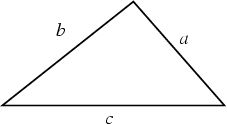Specifying three sides uniquely determines a Triangle whose Area is given by Heron's Formula,(1)

where(2)

is the Semiperimeter of the Triangle. Letbe the Circumradius, then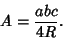(3)

Using the Law of Cosines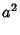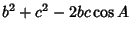(4)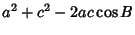(5)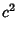(6)

gives the three Angles as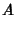(7)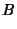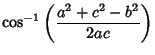(8)(9)

See also AAA Theorem, AAS Theorem, ASA Theorem, ASS Theorem, Heron's Formula, SAS Theorem, Semiperimeter, Triangle